# AP Statistics Multiple-Choice Practice Questions: Exploring Bivariate Data 1

### Test Information13 questions30 minutes

1. A study collects data on average combined SAT scores (math, critical reading, and writing) and percentage of students who took the exam at 100 randomly selected high schools. Following is part of the computer printout for regression: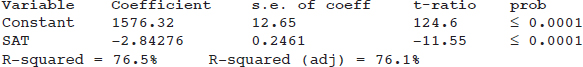Which of the following is a correct conclusion?

2. A simple random sample of 35 world-ranked chess players provides the following statistics:

Number of hours of study per day: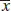= 6.2, sx = 1.3

Yearly winnings: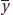= \$208,000, sy = \$42,000

Correlation r = 0.15

Based on this data, what is the resulting linear regression equation?

3. A rural college is considering constructing a windmill to generate electricity but is concerned over noise levels. A study is performed measuring noise levels (in decibels) at various distances (in feet) from the campus library, and a least squares regression line is calculated with a correlation of 0.74. Which of the following is a proper and most informative conclusion for an observation with a negative residual?

4. Consider the following three scatterplots: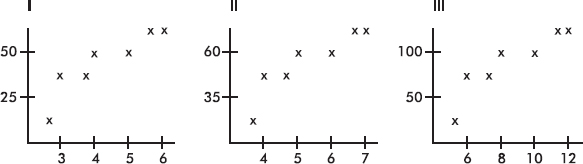Which has the greatest correlation coefficient?

5. Suppose the correlation is negative. Given two points from the scatterplot, which of the following is possible?

I.The first point has a larger x-value and a smaller y-value than the second point.

II.The first point has a larger x-value and a larger y-value than the second point.

III.The first point has a smaller x-value and a larger y-value than the second point.

6. Consider the following residual plot: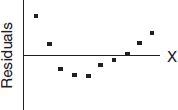Which of the following scatterplots could have resulted in the above residual plot? (The y-axis scales are not the same in the scatterplots as in the residual plot.)

7. Suppose the regression line for a set of data,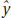= 3x + b, passes through the point (2, 5). Ifandare the sample means of the x- and y-values, respectively, then=

8. Suppose a study finds that the correlation coefficient relating family income to SAT scores is r = +1. Which of the following are proper conclusions?

I.Poverty causes low SAT scores.

II.Wealth causes high SAT scores.

III.There is a very strong association between family income and SAT scores.

9. A study of department chairperson ratings and student ratings of the performance of high school statistics teachers reports a correlation of r = 1.15 between the two ratings. From this information we can conclude that

10. Which of the following statements about correlation r is true?

11. Question below refers to the following:

The relationship between winning game proportions when facing the sun and when the sun is on one's back is analyzed for a random sample of 10 professional tennis players. The computer printout for regression is below: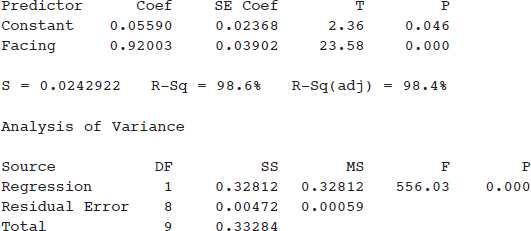What is the equation of the regression line, where facing and back are the winning game proportions when facing the sun and with back to the sun, respectively?

12. Question below refers to the following:

The relationship between winning game proportions when facing the sun and when the sun is on one's back is analyzed for a random sample of 10 professional tennis players. The computer printout for regression is below:What is the correlation?

13. Question below refers to the following:

The relationship between winning game proportions when facing the sun and when the sun is on one's back is analyzed for a random sample of 10 professional tennis players. The computer printout for regression is below:For one player, the winning game proportions were 0.55 and 0.59 for facing and back, respectively. What was the associated residual?